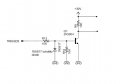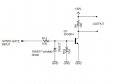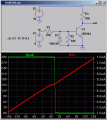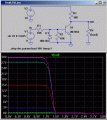# help with transistor switching

#### minkey01

Joined Jul 23, 2014
185
Hi. Could someone review this circuit and let me know if it will work as I intend? Questions below :

1) The trigger will sometimes be connected to different sources ranging from -15V DC to +15V DC. Is this ok? Can the circuit handle this range and will it still trigger throughout that entire range (everything except 0V)?

2) Is that 30V too much? How much can I put through that transistor?

3) Do I have the wiring right? I want the output of 30V to be turned off and on by the trigger.

4) Are my component values right?

Let me know what you think. Thanks so much for the help!#### jjw

Joined Dec 24, 2013
818
1) Output is ~ 0V when trigger is from about +1.8V to +15V. Otherwise +30V
2) 40V is absolute maximum
3) Wiring is ok
4) Values are ok, R9 might need adjustment depending on the circuit connected to it.

#### crutschow

Joined Mar 14, 2008
33,348
To elaborate slightly on jjw's post --

1)For that circuit the transistor will be fully on when the trigger voltage is above about 2V and fully off when the trigger voltage is 0V or less.
It will stay off when the voltage is negative.
If you want the transistor to be on for either a positive or negative input, then you will need to modify the circuit. I have a circuit using a couple added transistors that will work, if that's what you need.

2) 30V is fine for that transistor. It's rated for 40V maximum.

3,4) The circuit and component values look okay, with the limitations in operation I noted in 1).

#### minkey01

Joined Jul 23, 2014
185
thanks guys.

but JJW is saying the ouput is +30V when then transistor is off and +0V when it is on.
Crutschow is saying the opposite.

hmmmm...if the trigger is 0V, I would think the transistor is off and that output at R9 would be 0V. JJW, are you sure?

Ok. I don't mind the negative trigger volts not doing anything. We can leave it, as long as it won't damage anything?

What is the max trigger +V for this transistor?

What are the different reasons R9 should be adjusted?

Thanks for the help on the details!!

#### Bordodynov

Joined May 20, 2015
3,116

#### jjw

Joined Dec 24, 2013
818
thanks guys.

but JJW is saying the ouput is +30V when then transistor is off and +0V when it is on.
Crutschow is saying the opposite

hmmmm...if the trigger is 0V, I would think the transistor is off and that output at R9 would be 0V. JJW, are you sure?

............................................................

What are the different reasons R9 should be adjusted?

Thanks for the help on the details!!
No, Crutschow says the same thing: when voltage is above 2V, the transistor is fully conducting and it pulls the output to about 0.2V
When transistor is off, no current flows through R9 and the output is 30V.
If the output is connected for example to a 10kohm resistor the output swing would be 0-15V.
If about 30V swing is needed, R9 should be much smaller and also the base current of the transistor should be increased.

#### minkey01

Joined Jul 23, 2014
185
No, Crutschow says the same thing: when voltage is above 2V, the transistor is fully conducting and it pulls the output to about 0.2V
When transistor is off, no current flows through R9 and the output is 30V.
If the output is connected for example to a 10kohm resistor the output swing would be 0-15V.
If about 30V swing is needed, R9 should be much smaller and also the base current of the transistor should be increased.
If no current is flowing through R9, how can the output be 30V?

Ohms law say V=IR. V= 0 x 10,000 = 0#### jjw

Joined Dec 24, 2013
818
I am talking about voltage between R9 and ground.
Apparently you mean voltage over R9 ?

#### minkey01

Joined Jul 23, 2014
185
I am talking about voltage between R9 and ground.
Apparently you mean voltage over R9 ?
yes. i think i want across R9. i'm trying to turn 30V off and on. this is the correct way to do it?

thanks.

#### AnalogKid

Joined Aug 1, 2013
10,785
If no current is flowing through R9, how can the output be 30V?
Ohms law say V=IR. V= 0 x 10,000 = 0
Correct, but that 0 V is the voltage *across R9 only*, not the voltage from either end of R9 to anywhere else.

30 V (top of R9) - 0 V (voltage drop across R9) = 30 V (bottom of R9). Something to remember, Ohm's Law is a very local calculation. It relates the voltage across a part to the current through that part only. Extending this to voltages and currents somewhere else depends on the circuit, device physics, and many other details. So the output with the transistor off is 30 V with respect to GND, and 0 V with respect to the other end of the load.

ak

•Sinus23

#### jjw

Joined Dec 24, 2013
818
yes. i think i want across R9. i'm trying to turn 30V off and on. this is the correct way to do it?

thanks.
What do you want to do?
Switch 30V supply voltage to some device or have some trigger signal 0-30V, which you now have between R9 and gnd ?

#### minkey01

Joined Jul 23, 2014
185
What do you want to do?
Switch 30V supply voltage to some device or have some trigger signal 0-30V, which you now have between R9 and gnd ?

why is this getting so confusing? lol

this is a simple transistor switch circuit.

this is what i want:

when the SYNTH GATE INPUT is 0V then the transistor should not be activated and therefore 0V across R9 (marked OUTPUT)

when the SYNTH GATE INPUT is 15V then the transistor should be activated and therefore 30V across R9 (marked OUTPUT)

#### minkey01

Joined Jul 23, 2014
185
Correct, but that 0 V is the voltage *across R9 only*, not the voltage from either end of R9 to anywhere else.

30 V (top of R9) - 0 V (voltage drop across R9) = 30 V (bottom of R9). Something to remember, Ohm's Law is a very local calculation. It relates the voltage across a part to the current through that part only. Extending this to voltages and currents somewhere else depends on the circuit, device physics, and many other details. So the output with the transistor off is 30 V with respect to GND, and 0 V with respect to the other end of the load.

ak
i am only worried about the area marked output (across R9), because i'm using this as the output.

if my output was wired differently, yes there would be different results, but that is not the circuit.

#### jjw

Joined Dec 24, 2013
818
why is this getting so confusing? lol
this is a simple transistor switch circuit.
this is what i want:
when the SYNTH GATE INPUT is 0V then the transistor should not be activated and therefore 0V across R9 (marked OUTPUT)
Yes, the circuit works as you want, there is 0V or 30V across R9 or in other words 30V or 0V between R9 and gnd.

#### MikeML

Joined Oct 2, 2009
5,444
If the output is not loaded at all, here is a sweep of the output vs the input voltage. Note the "trip point" around 1.5V.The bigger question is what happens when you load the output. Here is V(out) for values of load resistance = 100, 1K, 10K, 100K and 1megΩ:•cmartinez

#### cmartinez

Joined Jan 17, 2007
8,122
If the output is not loaded at all, here is a sweep of the output vs the input voltage. Note the "trip point" around 1.5V.

View attachment 93781

The bigger question is what happens when you load the output. Here is V(out) for values of load resistance = 100, 1K, 10K, 100K and 1megΩ:

View attachment 93782
Mike, could you post the .asc file for the second circuit? I'd like to see how you created a variable load at the output. Thanks!

#### AnalogKid

Joined Aug 1, 2013
10,785
i am only worried about the area marked output (across R9), because i'm using this as the output.

if my output was wired differently, yes there would be different results, but that is not the circuit.
The circuit I described and responded to is the one in your schematic. What I wrote describes the output of that circuit. If you don't see that, then I'll try again.

ak

#### ScottWang

Joined Aug 23, 2012
7,389
What is the max trigger +V for this transistor?
Ic=(30V-(Vce_Q1)_/R9
Ic=(30V-0.2V)/10K
Ic=2.98mA

According to the datasheet the Vce get into the saturation status then hFE = 10, Ib=Ic/10=2.98mA=0.298mA, the Ib is not enough 1mA, so maybe the bjt won't get into the saturation status.

Assuming that the Ic =50mA, Ib=50mA/10=5mA, Vbe=0.95V, V_R13=5mA * R13 = 5mA*10K = 50V
I_R10=0.95V/R10=0.95V/10K=95uA, so ignore it.

Vin max = 50V+0.95V=50.9V.

#### AnalogKid

Joined Aug 1, 2013
10,785
why is this getting so confusing? lol
this is a simple transistor switch circuit.
this is what i want:
when the SYNTH GATE INPUT is 0V then the transistor should not be activated and therefore 0V across R9 (marked OUTPUT)
when the SYNTH GATE INPUT is 15V then the transistor should be activated and therefore 30V across R9 (marked OUTPUT)
That is exactly what your circuit does, up to the max collector current for the transistor.
Why is this so confusing?

ak

Last edited: